机器学习基础算法(3)数据降维，数据的划分以及转换器和估计器1411-李同学

, ,

数据降维

• 特征选择

• 主成分分析

特征选择

• 冗余：部分特征相关度高，容易消耗计算性能

• 噪声：部分特征对预测结果有影响

• filter 过滤式 variance threshold

• embedded 嵌入式 正则化、决策树

• wrapper 包裹式

过滤式特征选择

sklearn.feature_selection.VarianceThreshold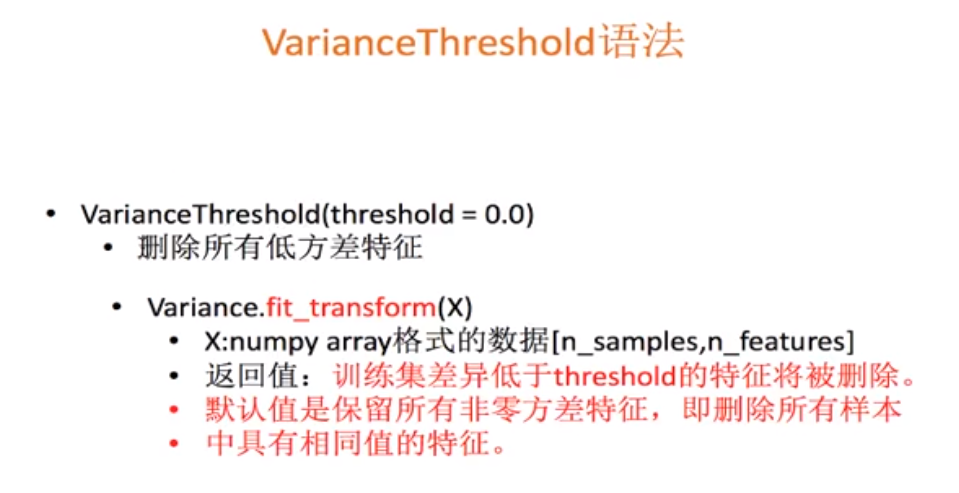代码演示

from sklearn.feature_selection import VarianceThreshold

def var():
#特征选择
var=VarianceThreshold(threshold=1.0) #取值根据实际的需求
data=var.fit_transform([[0,2,0,3],[0,1,4,3],[0,1,1,3]])
print(data)

if __name__=="__main__":
var()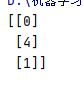主程序分析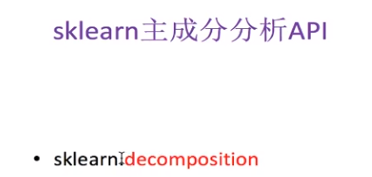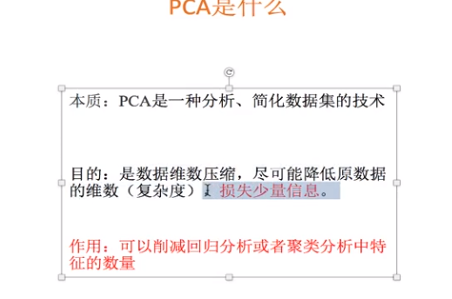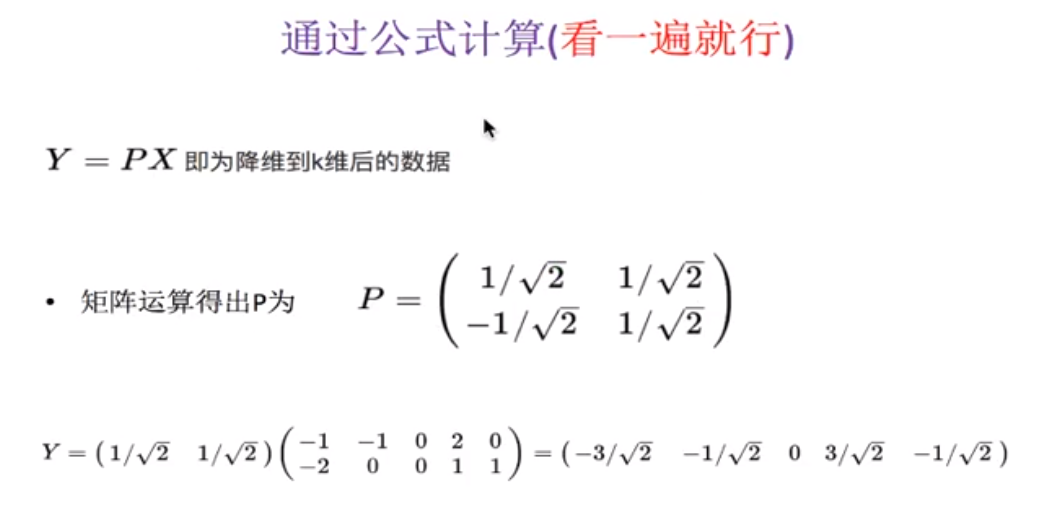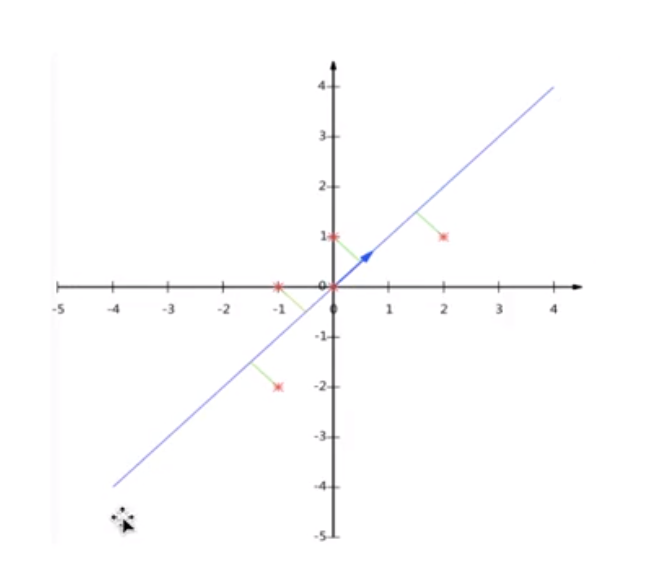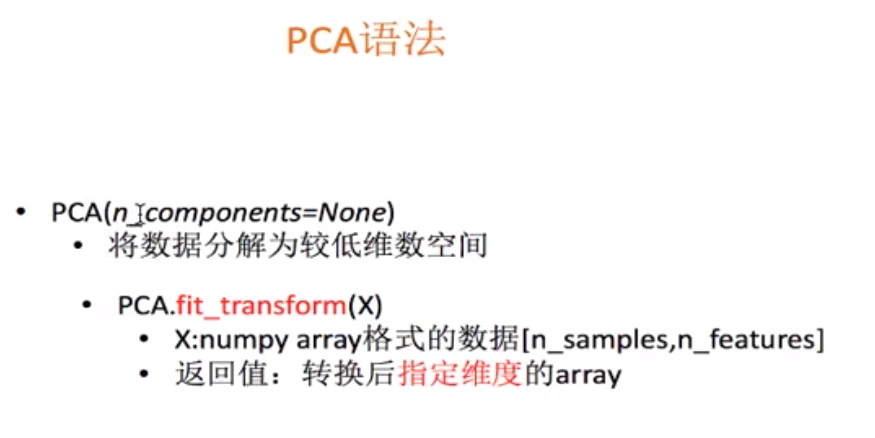n_components:

• 小数 百分比（0~1）保留多少信息 一般取90~95%

• 整数 减少到的特征数量

pca代码

from sklearn.decomposition import PCA

def pca():
#主成分分析进行特征降维
pca=PCA(n_components=0.9)

data=pca.fit_transform([[2,8,4,5],[6,3,0,8],[5,4,9,1]])

print(data)

if __name__=="__main__":
pca()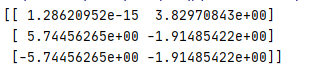降维案例01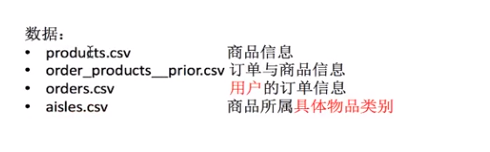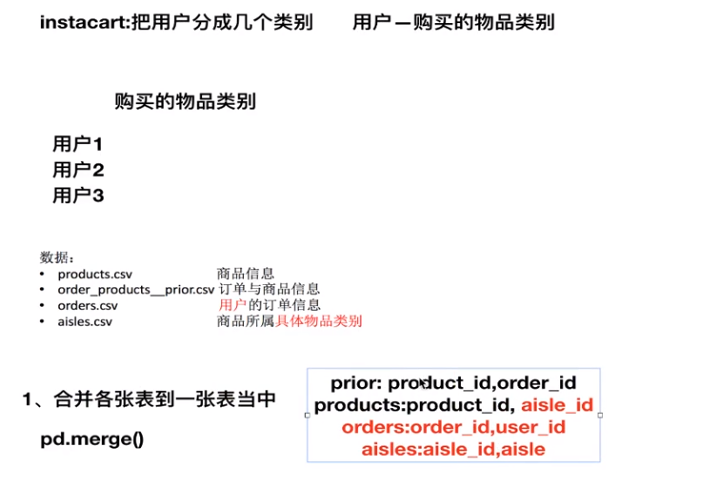降维案例

import pandas as pd
from sklearn.decomposition import PCA

#读取4张表

#合并四张表到一张表当中 （用户-物品类别）
_mg=pd.merge(prior,products,on=["product_id","product_id"])
_mg=pd.merge(_mg, orders,on=["order_id","order_id"])
mt=pd.merge(_mg,aisles,on=["aisle_id","aisle_id"])

#建立行和列
# 交叉表 特殊的分组工具
cross=pd.crosstab(mt["user_id"],mt["aisle"])

#进行主成分分析
pca=PCA(n_components=0.9)
data=pca.fit_transform(cross)
print(data)
1、合并表找到order_id 和 aisle_id的关系
2、用交叉表转换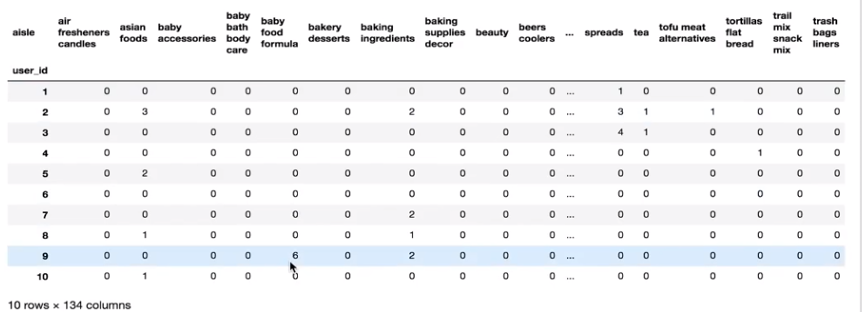3、主成分分析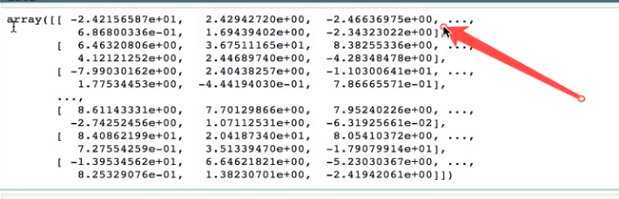机器学习算法分类以及开发流程

• 学会分析问题，用算法解决问题

• 掌握算法基本思想

• 利用库和框架解决问题

算法分类

1、数据类型

• 离散型数据：由记录不同类别个体的数目所得到的数据，又称计数数据，不能再细分，也不能进一步地提高精确度。

• 连续型数据：可以在某个范围内取任意数，即变量的取值可以是连续的，如长度时间质量值等。通常是非整数，含有小数部分。

2、 算法分类

1） 监督学习（预测）特征值+目标值

• 分类 k-近邻算法、贝叶斯分类、决策树与随机森林、逻辑回归、神经网络

• 回归 线性回归 岭回归

• 标注 隐马尔科夫模型 不做要求

2） 无监督学习 特征值无标签，无标准答案

• 聚类 k-means

1、明天天气多少度：回归

2、明天天气是阴、晴还是雨：分类

3、人脸年龄预测：回归

4、人脸识别：分类

开发流程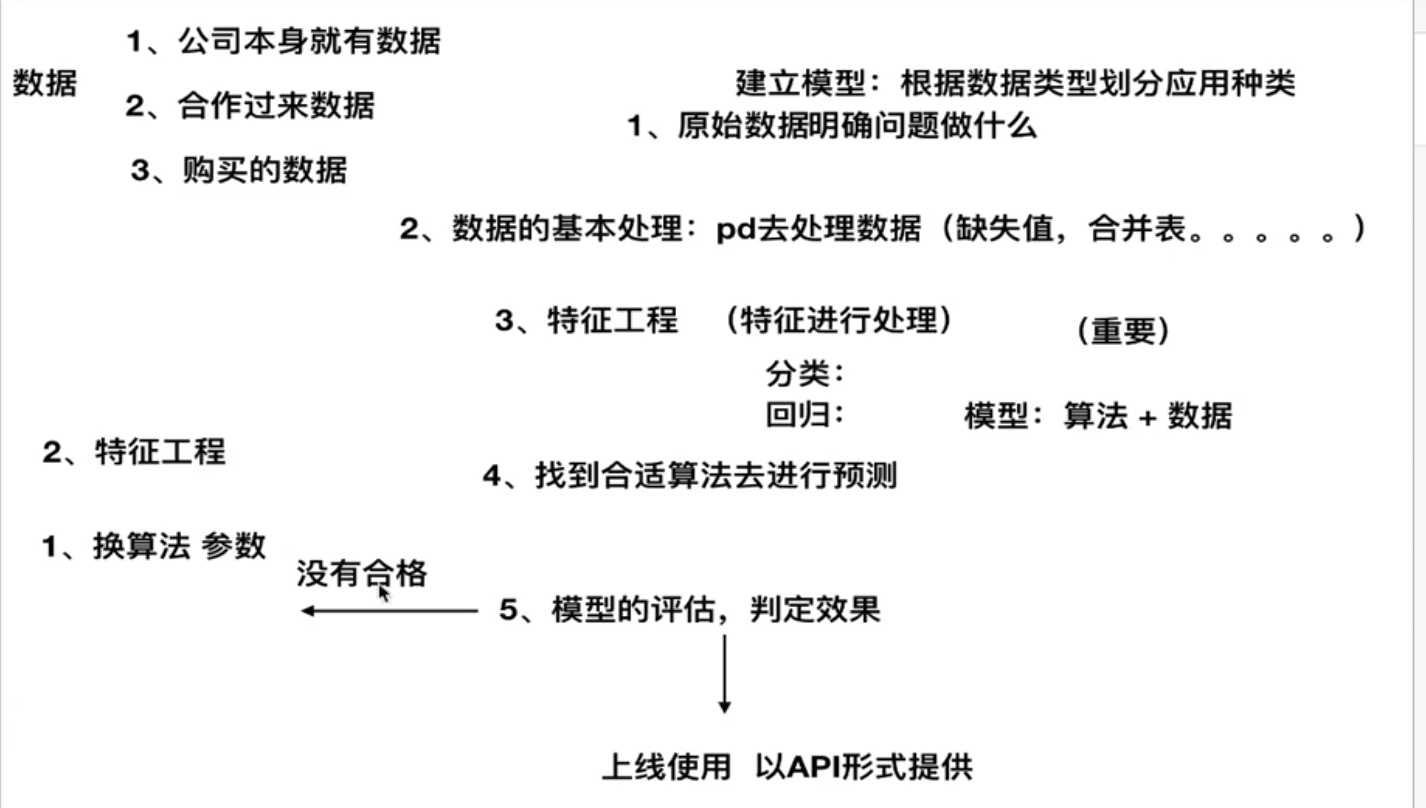数据的划分和介绍

1、sklearn 数据集

数据集划分

• 训练集 占大多数 70% 80% 75% 构建模型

• 测试集 30% 20% 25% 用于评估模型是否有效

sklearn.model_selection.train_test_split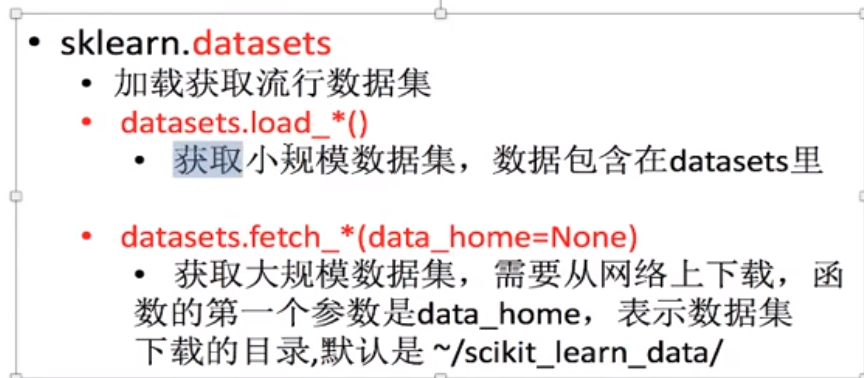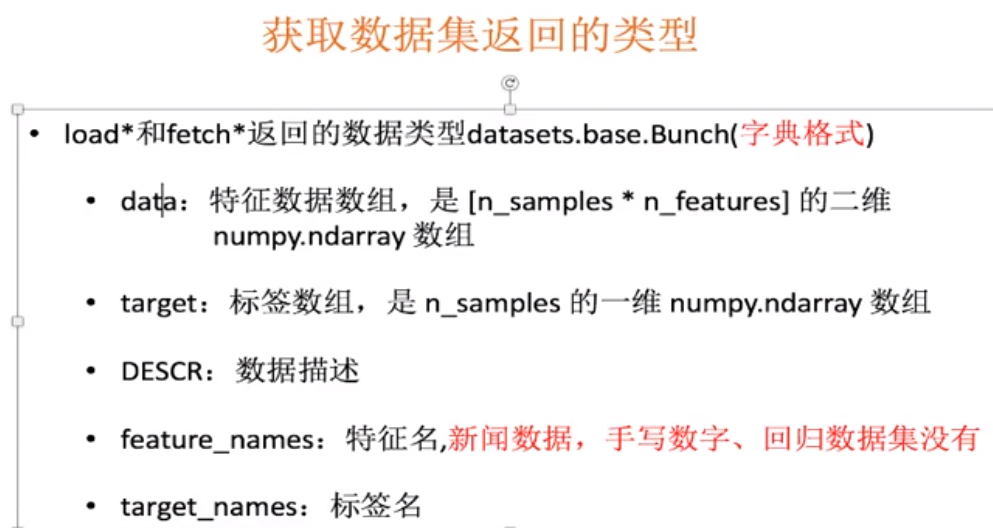获取鸢尾花 分类数据集 离散型

print("获取特征值")
print(li.data)
print("获取目标值")
print(li.target)
print(li.DESCR)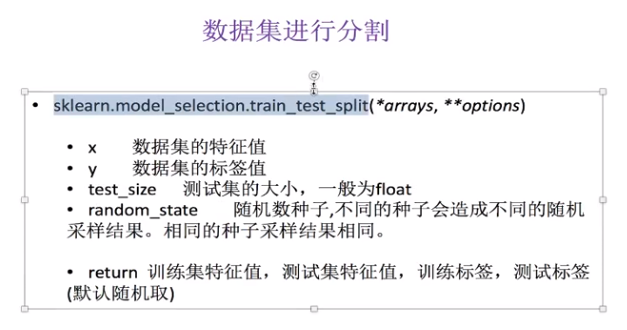数据集分割实例(1)

from sklearn.model_selection import train_test_split
# print("获取特征值")
# print(li.data)
# print("获取目标值")
# print(li.target)
# print(li.DESCR)

#注意返回值，训练集  train x_train  y_train 测试集 test  x_test y_test
x_train,x_test,y_train, y_test=train_test_split(li.data,li.target,test_size=0.25)
print("训练集特征值和目标值：",x_train,y_train)
print("测试集特征值和目标值：",x_test,y_test)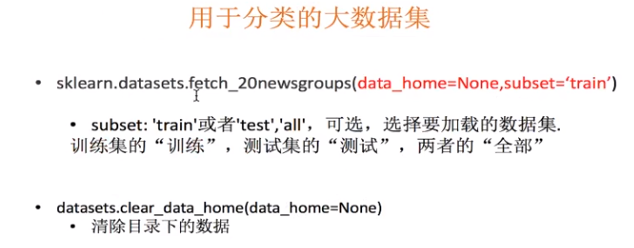分类数据集实例（2）

news=fetch_20newsgroups(subset="all")
print(news.data)
print(news.target)

回归数据集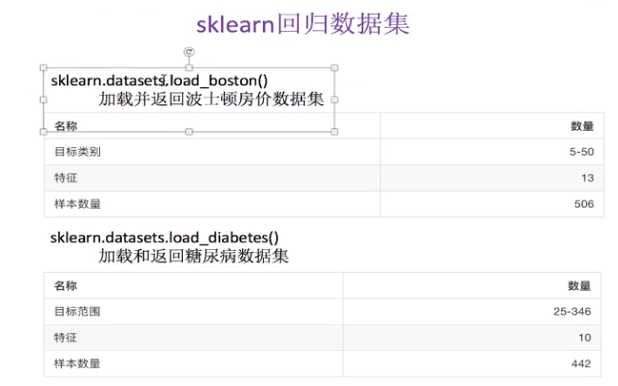回归数据集实例

print("获取特征值")
print(lb.data)
print("获取目标值")
print(lb.target)
print(lb.DESCR)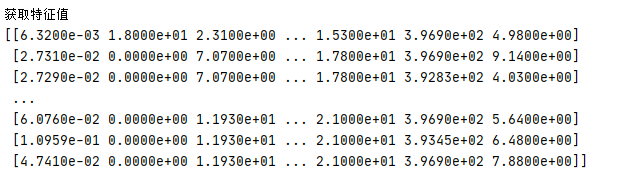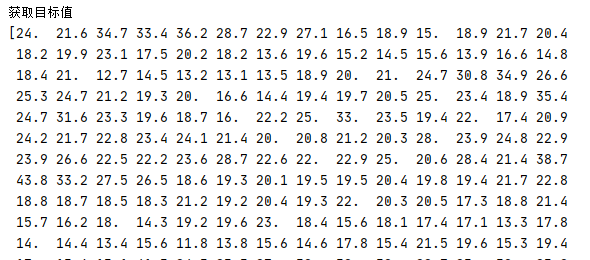转换器与估计器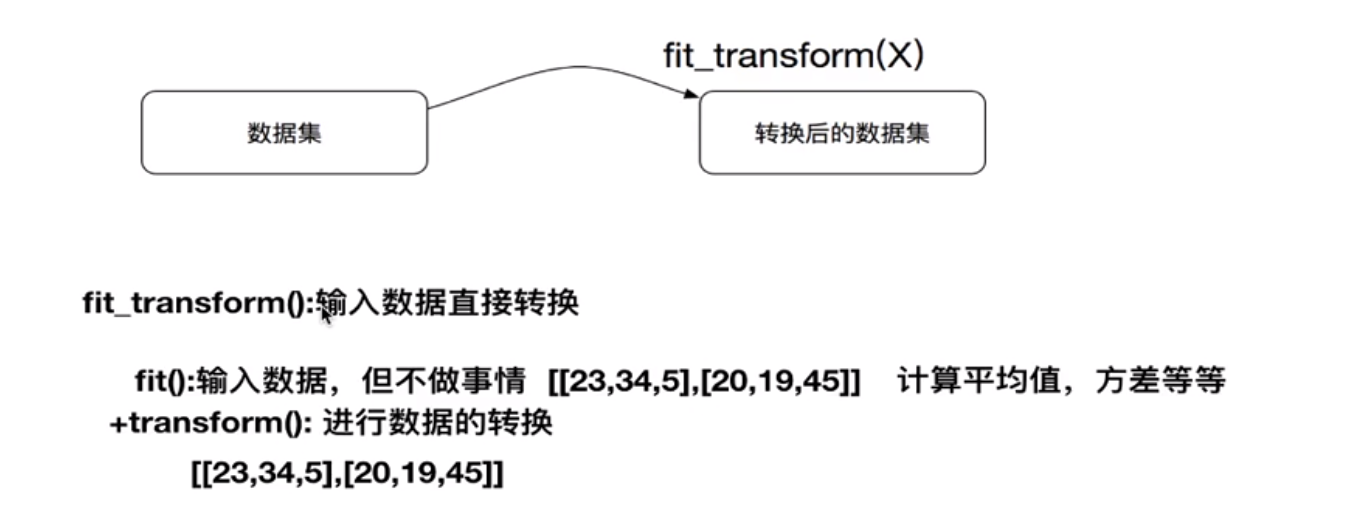fit部分计算输入的数据的平均值和标准差，transform将结果带入数据中。

估计器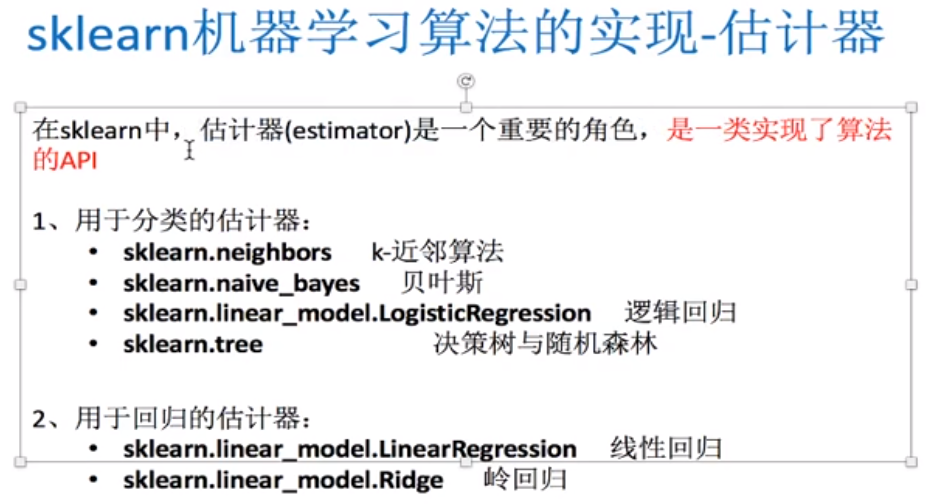估计器操作流程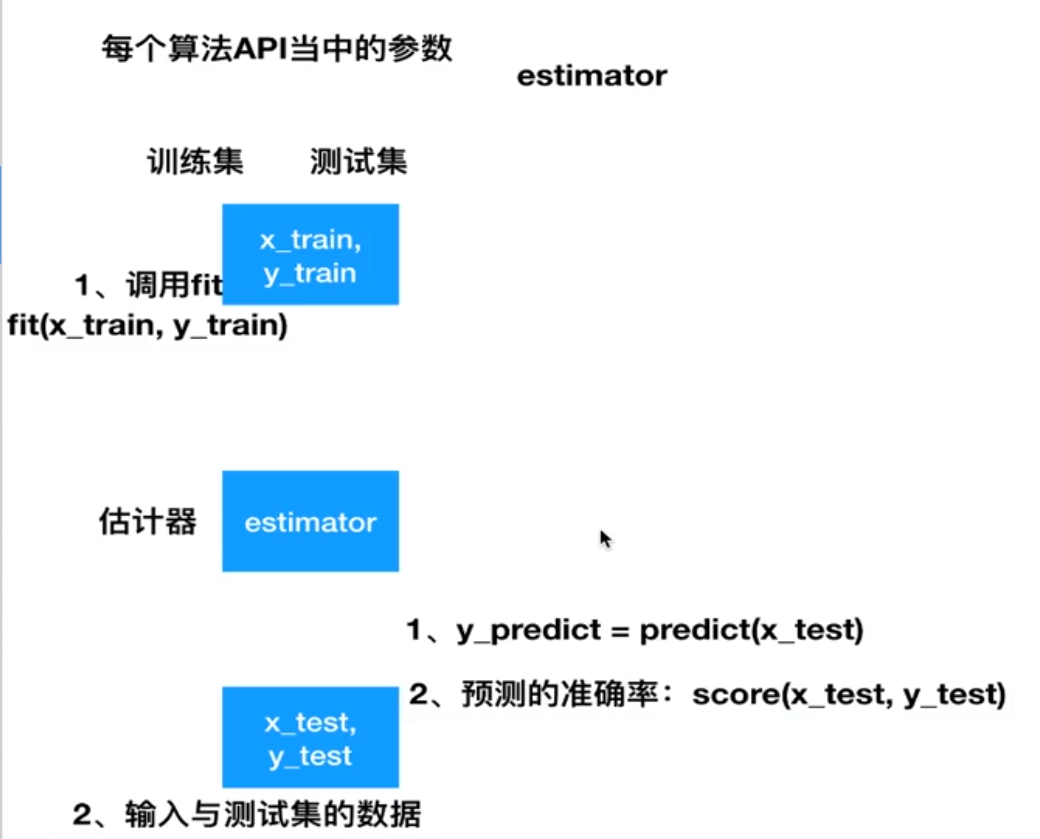评论 抢沙发觉得文章有用就打赏一下文章作者

支付宝扫一扫打赏微信扫一扫打赏Vieu3.3主题Q Q 登 录